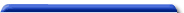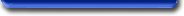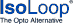IsolatorsIsolator Part Numbering

The following table shows the IsoLoop part numbering system and contains links to selector guides:

IL  11  T A E  TR7
 Product Family    IL = Isolators Base Part Number    2 = 5-Channel Digital Input    4 = Industry-Std Transceiver    5 = 2 Mbps, DC-Correct    6 = Passive Input    7 = 110 Mbps, Digital Input    8 = 110 Mbps, DC-Correct    30 = Digital-In, 4 Mbps Xcvr    31 = Passive-In, 5 Mbps Xcvr    32 = Passive-In, 1/8-Load Xcvr    34 = Passive-In, 20 Mbps Xcvr    35 = Digital-In, 40 Mbps Xcvr    36 = PROFIBUS Transceiver Channel Configuration    10 = 1 Channel    11 = 2 Transmit    12 = 1 Xmit; 1 Receive    13 = 3 Transmit    14 = 2 Xmit; 1 Receive    15 = 4 Transmit    16 = 2 Xmit; 2 Receive    17 = 3 Xmit; 1 Receive    21 = 1 Receive; 1 Xmit    22 = RS-422    60 = 5 Transmit    61 = 4 Xmit; 1 Receive    62 = 3 Xmit; 2 Receive    85 = RS-485    1050 = CAN Variant    Blank = Standard    A = Open Drain Output    S = High Speed (150 Mbps)    T = High Temperature (125°C)    V = High Isolation Voltage    W = RS-485 Handshake Die Revision    (A, B, C, etc., if applicable) Package Size     -1 = MSOP or QSOP     -2 = PDIP     -3 = 0.15'' SOIC     -5 = Bare die     Blank = 0.3'' SOIC Package Materials    Blank = Lead (Pb)    E = RoHS Compliant Bulk Packaging    Blank = Tube    TR7 = 7'' Tape and Reel    TR13 = 13'' Tape and Reel